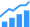# Facilitating High Level Synthesis from MATLAB generated C C++

Use case demonstrates that SLX for improving performance of an HLS implementation of this MATLAB generated code.MATLAB is the go-to toolbox for high level algorithm design in many application domains, ranging from signal processing to control systems and data analysis. MATLAB Coder generates executable C/C++ code from MATLAB implementations. However, the performance requirements of these applications often mandate a hardware implementation. SLX FPGA helps transform the auto-generated C/C++ code into a synthesizable, optimized, and hardware-aware implementation for high level synthesis (HLS).

To create a hardware implementation from a MATLAB design, we have two options: (1) Using MATLAB HDL CoderTM to generate a synthesizable HDL implementation or (2) Using MATLAB CoderTM to generate C/C++ code and passing it on to HLS tools that translate it into an HDL implementation. The first option is comparatively restricted in terms of the MATLAB functions, language constructs, and Simulink blocks supported by the HDL Coder. Moreover, it often requires hardware design expertise for tweaking and/or integrating the generated HDL code with components/features designed outside MATLAB. The second option is less restrictive with a much wider range of MATLAB functions and language constructs supported by the MATLAB C/C++ code generation tools; however, the generated code is not targeted for hardware implementation and often is not synthesizable. SLX FPGA is the perfect tool to bridge that gap.